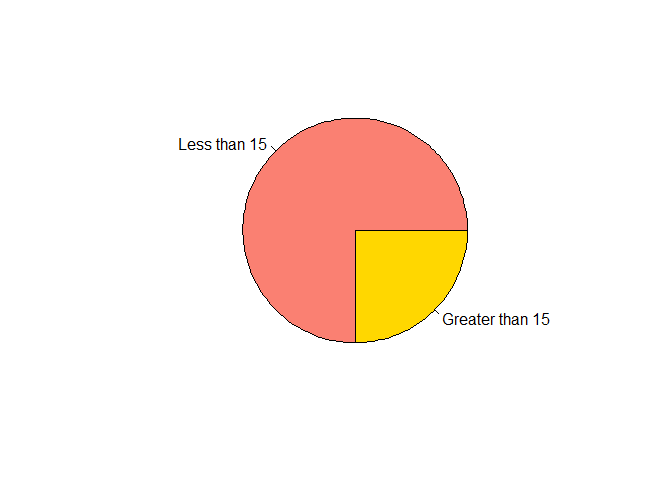# R for Beginners: Example Solutions of Simple Commands

Get the R solution file here.

## Problem 1

Use R as a calculator to compute the following values.

1. $$9 + 3(6 - 4)$$
9 + 3 * (6 - 4)
##  15
1. $$\sqrt{\frac{900}{12}}$$
sqrt(900 / 12)
##  8.660254
1. $$ln(14^2)$$, where $$ln$$ is the natural logarithm.
log(14^2)
##  5.278115
1. $$e(1)$$, which should give Euler’s number.
exp(1)
##  2.718282
1. Calculate the absolute value of $$13 - 22/1.2$$
abs(13 - 22 / 1.2)
##  5.333333

## Problem 2

Create the following vectors in R.

1. v1, which has the elements (5,7,3)
v1 <- c(5, 7, 3)
v1
##  5 7 3
1. v2, which has the elements (0,5,10,15,…,100)
v2 <- seq(0, 100, by = 5)
v2
##     0   5  10  15  20  25  30  35  40  45  50  55  60  65  70  75  80  85  90
##   95 100
1. v3, which has the elements (Yugoslavia,Yugoslavia,Canada,Canada,Czech Republic, Czech Republic,Croatia,Croatia,Hungary,Hungary,USA,USA)
v3 <- c("Yugoslavia", "Yugoslavia", "Canada", "Canada", "Czech Republic",
"Czech Republic", "Croatia", "Croatia", "Hungary", "Hungary", "USA", "USA")
v3
##   "Yugoslavia"     "Yugoslavia"     "Canada"         "Canada"
##   "Czech Republic" "Czech Republic" "Croatia"        "Croatia"
##   "Hungary"        "Hungary"        "USA"            "USA"
1. v3.1, which is the same as v3 above but uses the repeat command.
v3.1 <- rep(c("Yugoslavia", "Canada", "Czech Republic", "Croatia", "Hungary", "USA"),
each = 2)
v3.1
##   "Yugoslavia"     "Yugoslavia"     "Canada"         "Canada"
##   "Czech Republic" "Czech Republic" "Croatia"        "Croatia"
##   "Hungary"        "Hungary"        "USA"            "USA"
1. v4, which has the elements (2018,2012,2010,…,1918)
v4 <- c(2018, seq(2012, 1918, by = -2))
v4
##   2018 2012 2010 2008 2006 2004 2002 2000 1998 1996 1994 1992 1990 1988 1986
##  1984 1982 1980 1978 1976 1974 1972 1970 1968 1966 1964 1962 1960 1958 1956
##  1954 1952 1950 1948 1946 1944 1942 1940 1938 1936 1934 1932 1930 1928 1926
##  1924 1922 1920 1918
1. v5, a vector whose values start at 20, end at 0, and contains 36 evenly spaced elements
v5 <- seq(20, 0, length.out = 36)
v5
##   20.0000000 19.4285714 18.8571429 18.2857143 17.7142857 17.1428571
##   16.5714286 16.0000000 15.4285714 14.8571429 14.2857143 13.7142857
##  13.1428571 12.5714286 12.0000000 11.4285714 10.8571429 10.2857143
##   9.7142857  9.1428571  8.5714286  8.0000000  7.4285714  6.8571429
##   6.2857143  5.7142857  5.1428571  4.5714286  4.0000000  3.4285714
##   2.8571429  2.2857143  1.7142857  1.1428571  0.5714286  0.0000000

## Problem 3

For each of the following questions, use R to find the answer.

1. What are the 7th, 8th, and 12th elements of v5?
v5[c(7, 8, 12)]
##  16.57143 16.00000 13.71429
1. Which elements of v5 are less than or equal to 13.5?
which(v5 <= 13.5)
##   13 14 15 16 17 18 19 20 21 22 23 24 25 26 27 28 29 30 31 32 33 34 35 36
1. What is the value of each of the elements of v5 that is less than or equal to 13.5?
v5[v5 <= 13.5]
##   13.1428571 12.5714286 12.0000000 11.4285714 10.8571429 10.2857143
##    9.7142857  9.1428571  8.5714286  8.0000000  7.4285714  6.8571429
##   6.2857143  5.7142857  5.1428571  4.5714286  4.0000000  3.4285714
##   2.8571429  2.2857143  1.7142857  1.1428571  0.5714286  0.0000000
1. What is the percentage of elements of v5 that is less than 3.4?
mean(v5 < 3.4) * 100
##  16.66667
1. Which elements of v5 are greater than 10 and less than 13?
which(v5 > 10 & v5 < 13)
##  14 15 16 17 18
1. What are the values of the elements of v5 that are greater than 10 and less than 13?
v5[v5 > 10 & v5 < 13]
##  12.57143 12.00000 11.42857 10.85714 10.28571

## Problem 4

Draw a pie chart with two slices - the values of v5 that are greater than, and those that are less than 15. In other words, your pie chart should show the relative percentage of values in v5 that are greater than 15 versus those that are less than 15. Bonus point: make one of the pie slices the color salmon and the other slice the color gold.

slices <- table(v5 > 15)
lbls <- c("Less than 15", "Greater than 15")
pie(slices, labels = lbls, col = c("salmon", "gold"))## Problem 5

Find the following for the vector v1 (which you created above):

1. mean
mean(v1)
##  5
1. median
median(v1)
##  5
1. range
range(v1)
##  3 7
1. standard deviation
sd(v1)
##  2